CBSE Class 12 Sample Paper for 2018 Boards

Class 12
Solutions of Sample Papers and Past Year Papers - for Class 12 Boards

### Evaluate Definite Integral ∫ -2 to 2 (3x 2  - 2x + 4) as the limit of a sum.

This is a question of CBSE Sample Paper - Class 12 - 2017/18.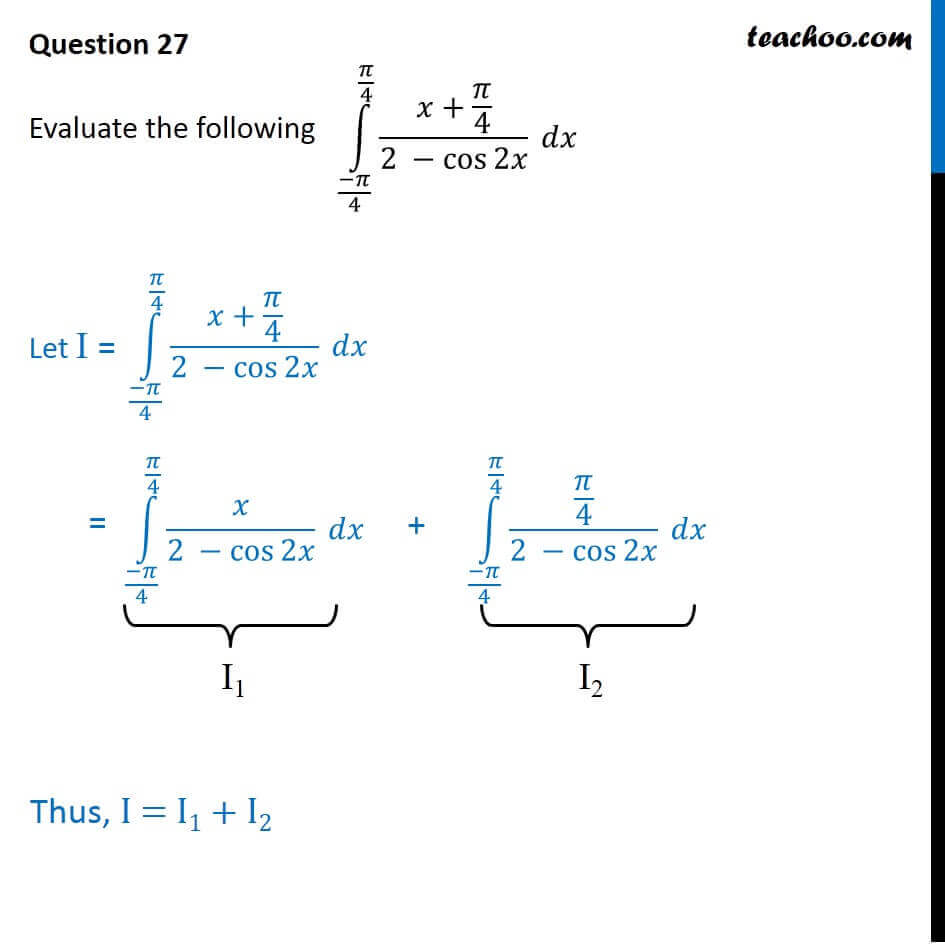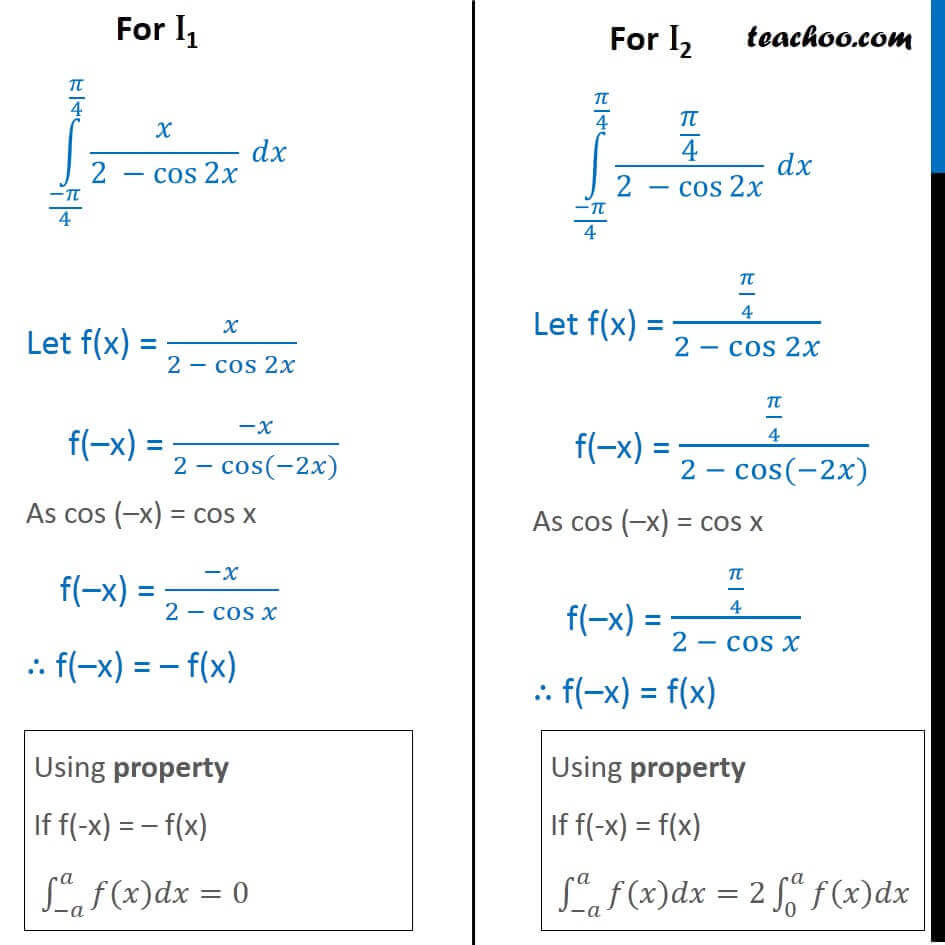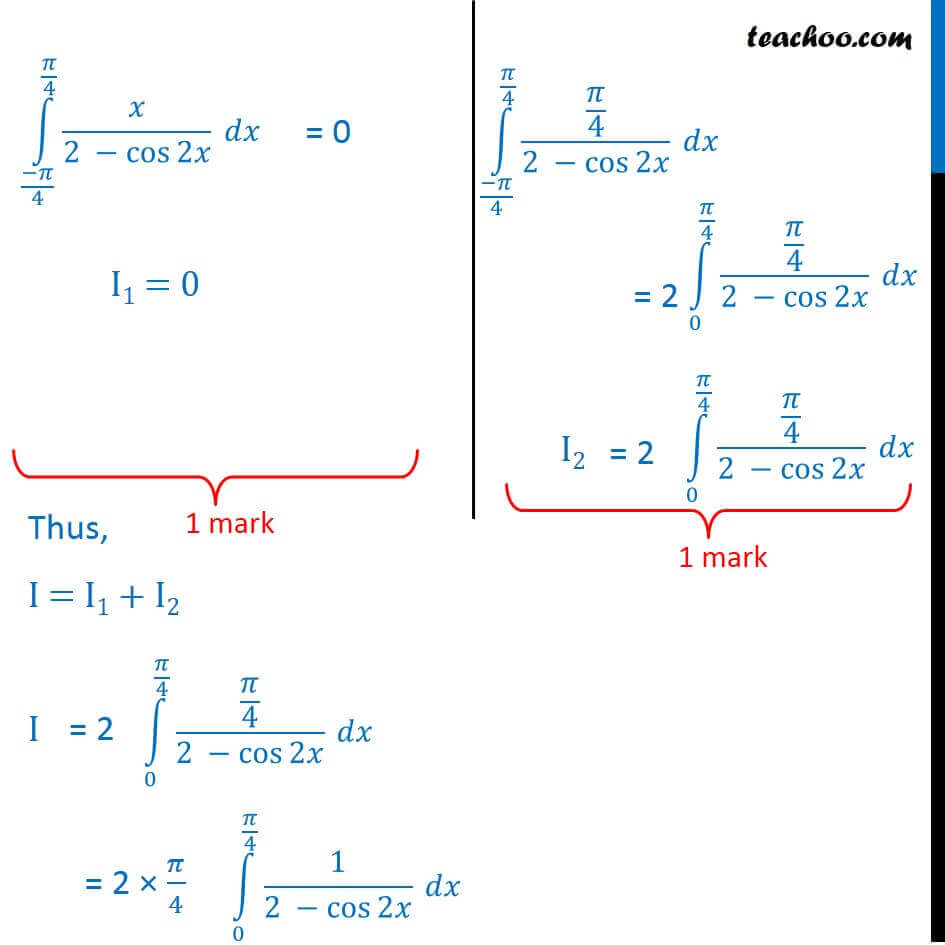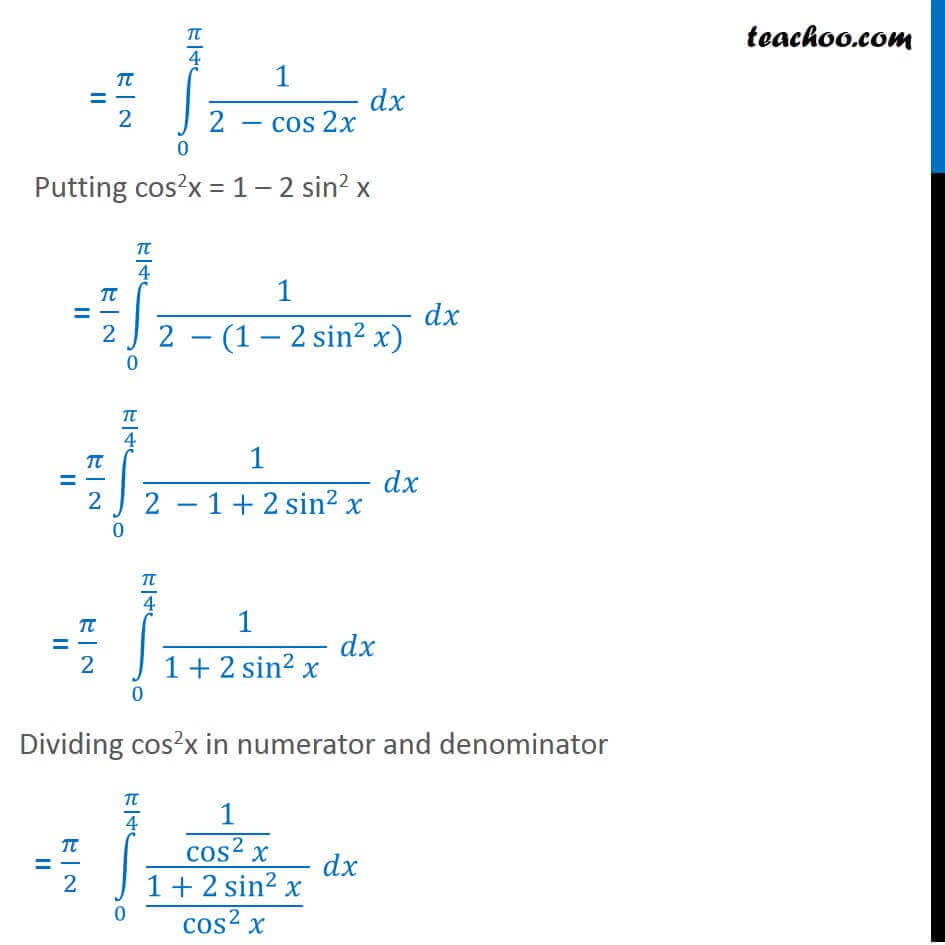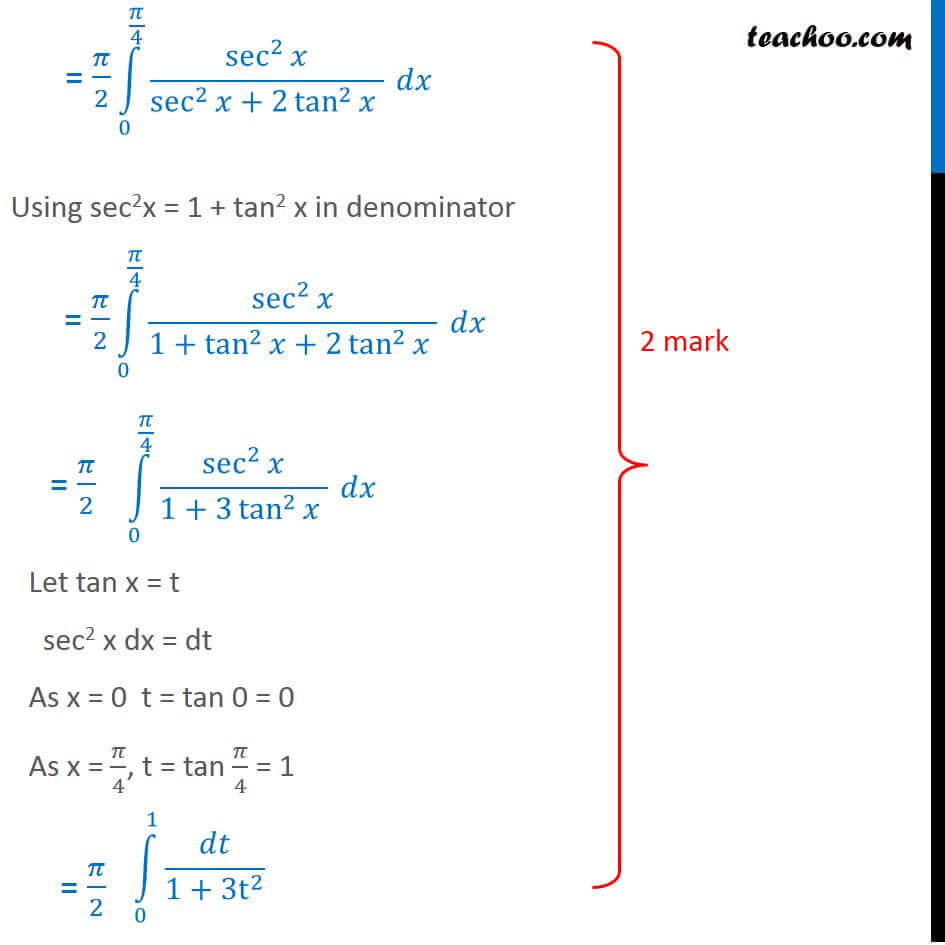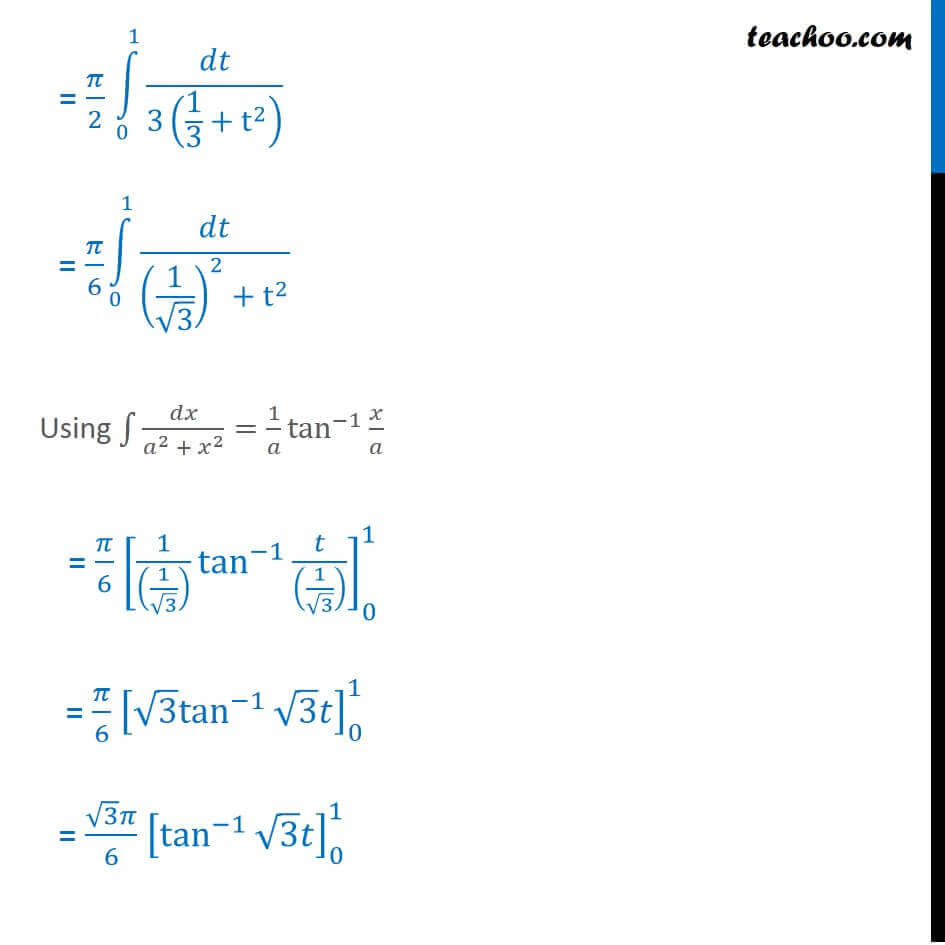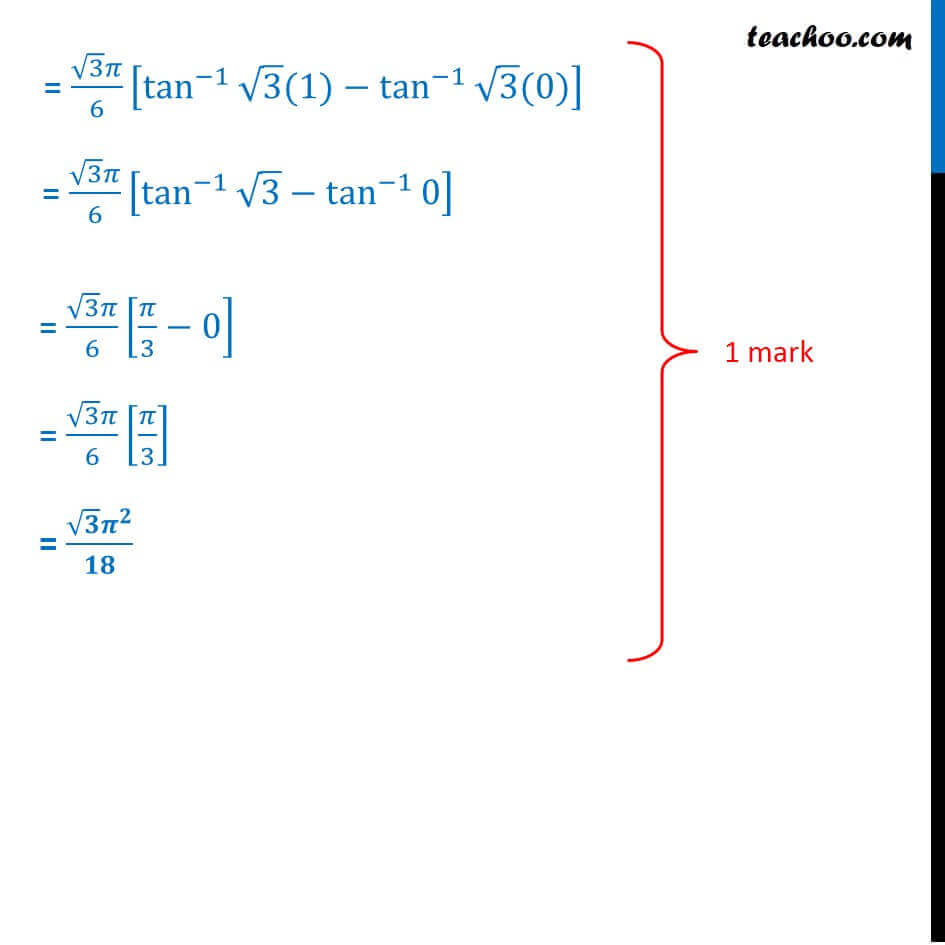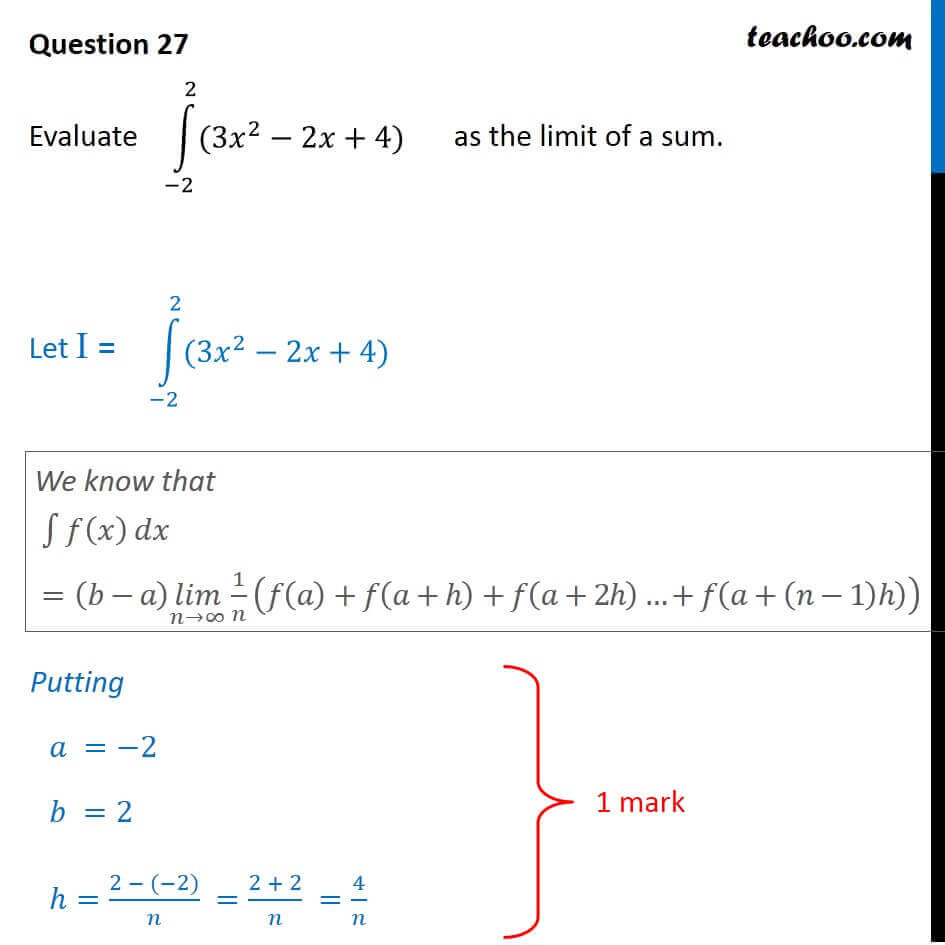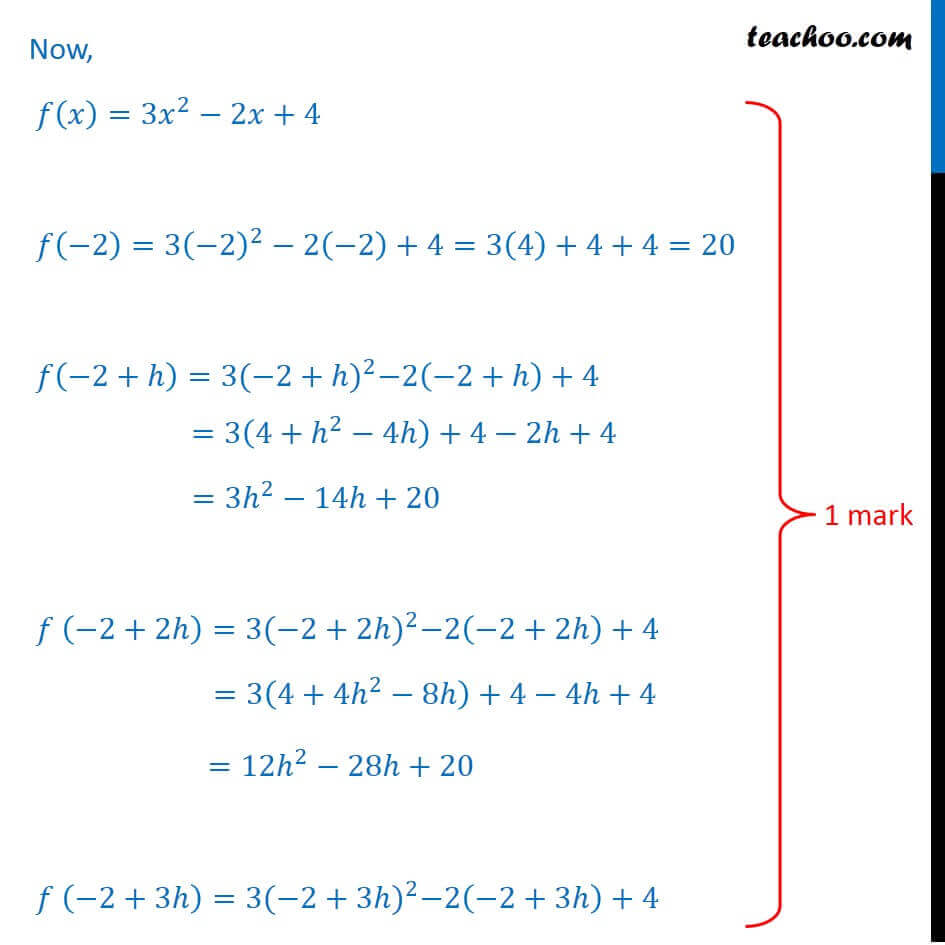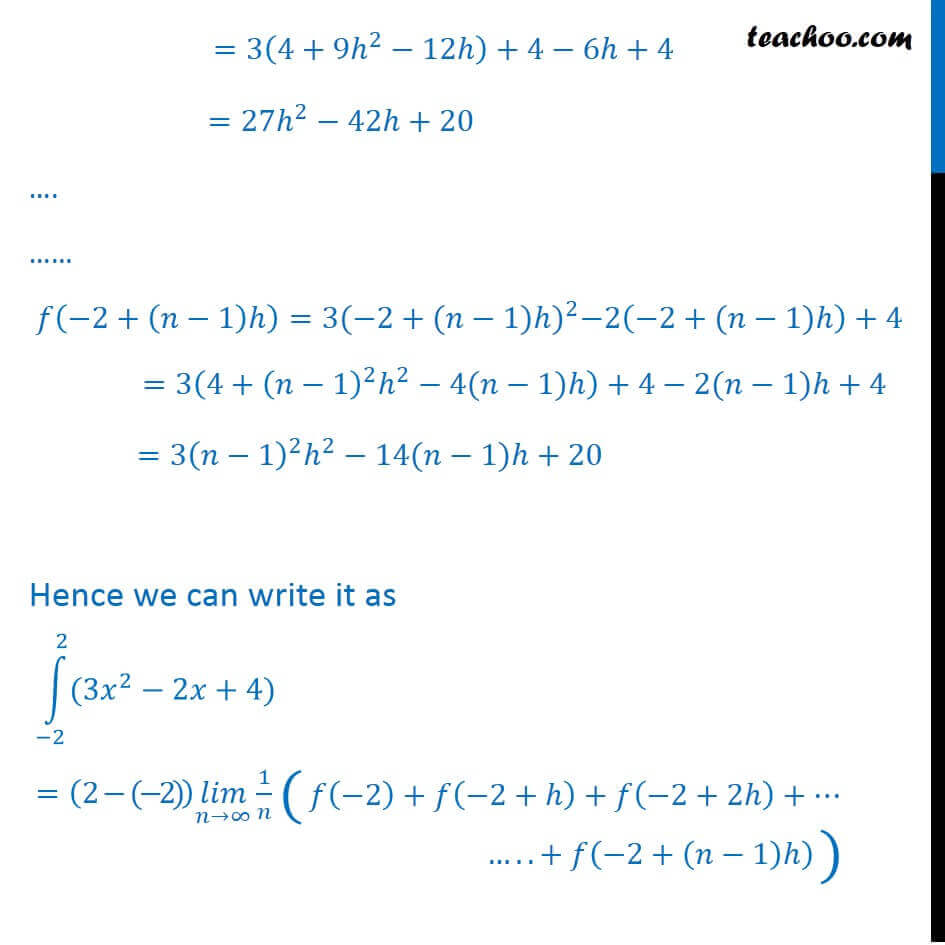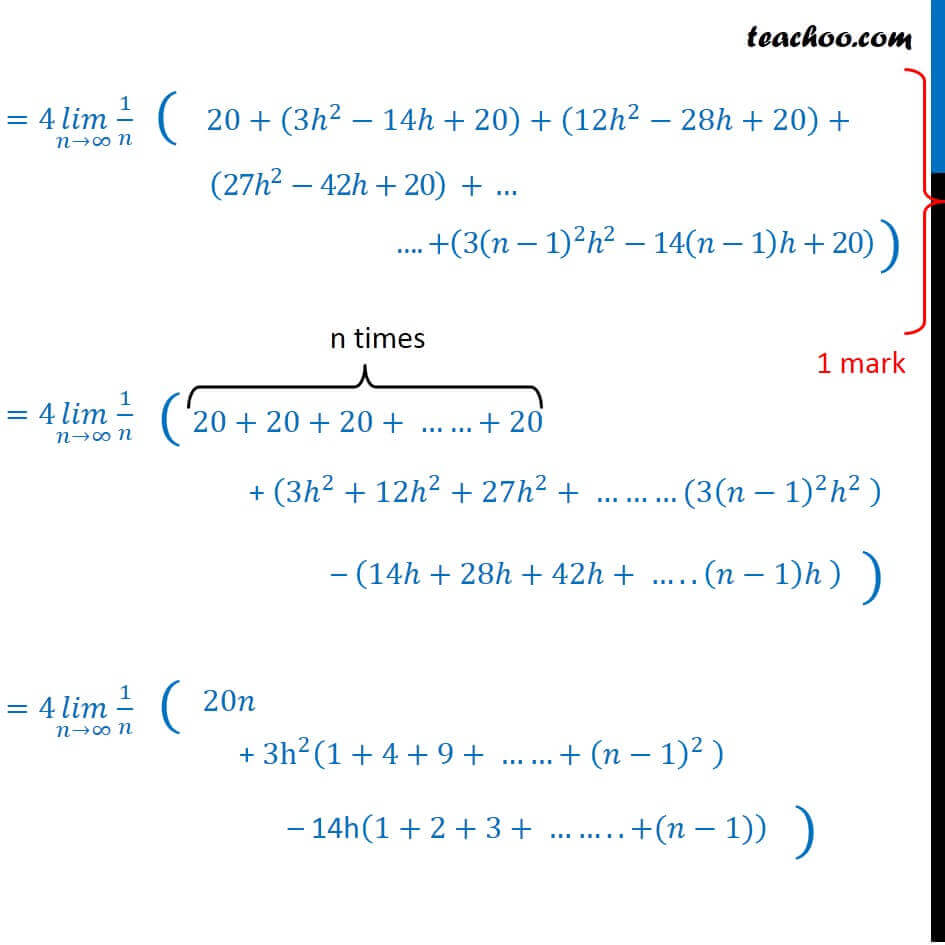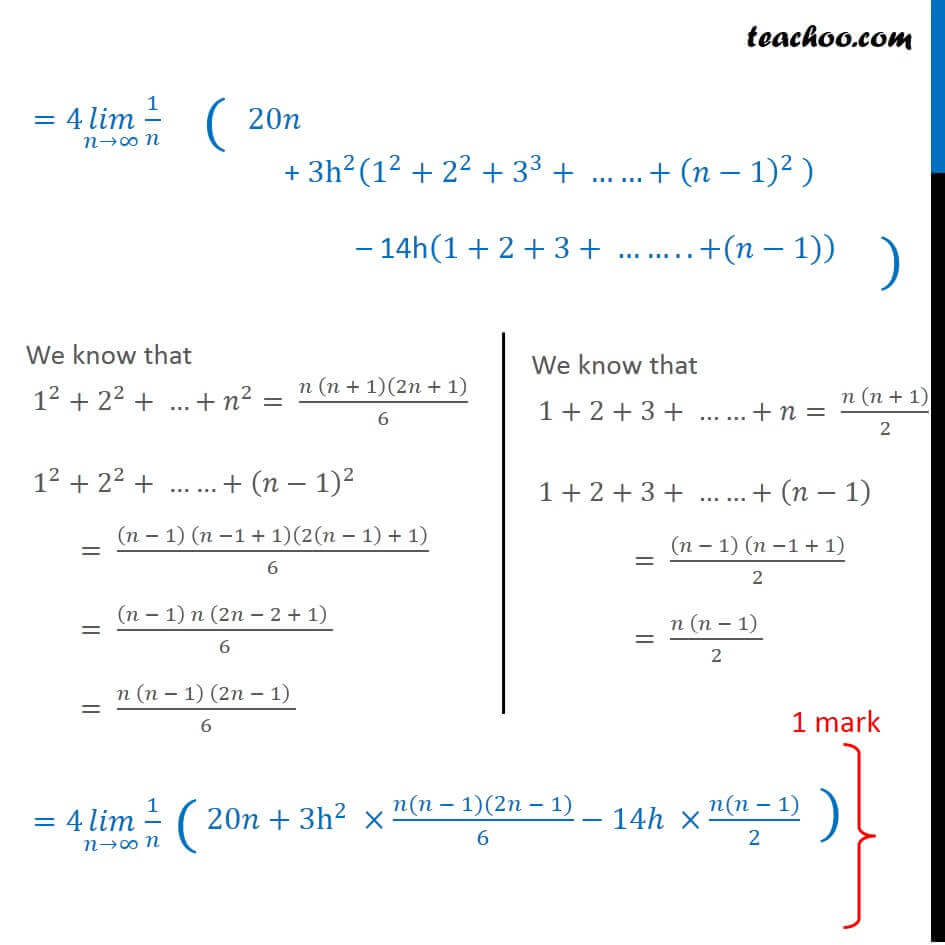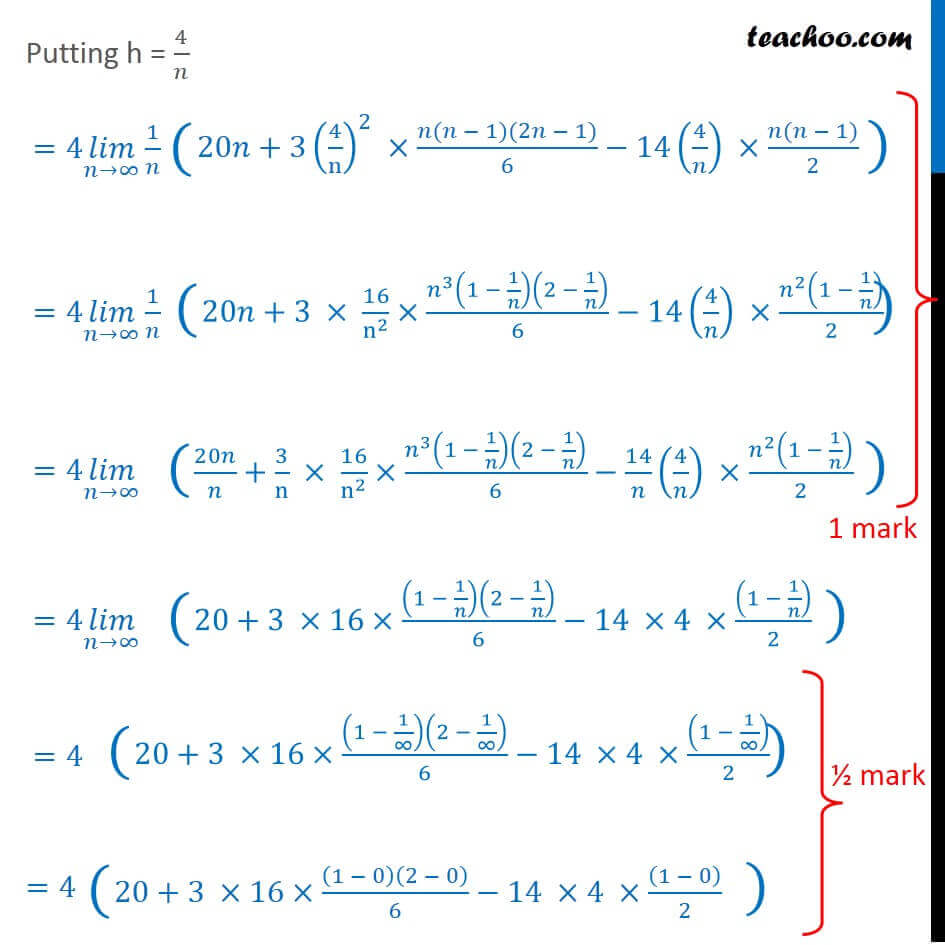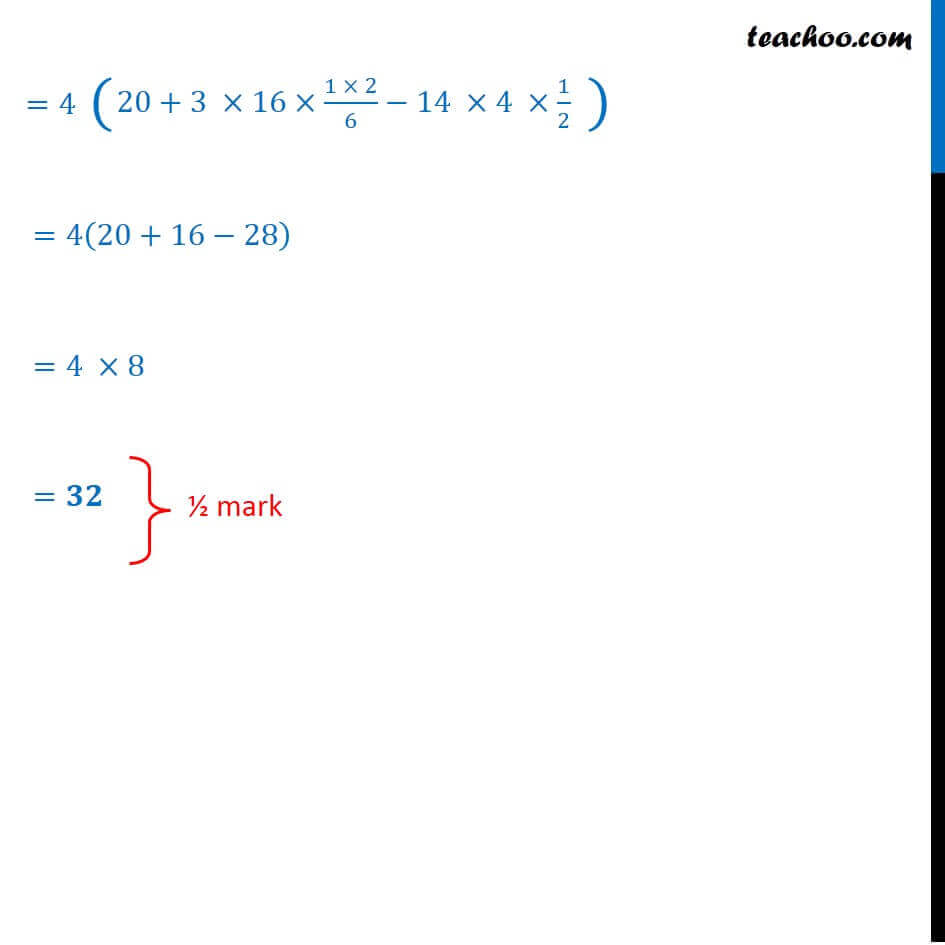### Transcript

Question 27 Evaluate the following 1_(( )/4)^( /4) ( + /4)/(2 cos 2 ) 1_(( )/4)^( /4) ( + /4)/(2 cos 2 ) 1_(( )/4)^( /4) /(2 cos 2 ) 1_(( )/4)^( /4) ( /4)/(2 cos 2 ) Let f(x) = /(2 cos 2 ) f( x) = ( )/(2 cos ( 2 ) ) As cos ( x) = cos x f( x) = ( )/(2 cos ) f( x) = f(x) Let f(x) = ( /4)/(2 cos 2 ) f( x) = ( /4)/(2 cos ( 2 ) ) As cos ( x) = cos x f( x) = ( /4)/(2 cos ) f( x) = f(x) Using property If f(-x) = f(x) _( )^ ( ) =0 Using property If f(-x) = f(x) _( )^ ( ) =2 _0^ ( ) 1_(( )/4)^( /4) /(2 cos 2 ) 1_(( )/4)^( /4) ( /4)/(2 cos 2 ) Thus, I = I1 + I2 I 1_0^( /4) ( /4)/(2 cos 2 ) 1_0^( /4) 1/(2 cos 2 ) 1_0^( /4) 1/(2 (1 2 sin^2 ) ) 1_0^( /4) 1/(1+2 sin^2 ) 1_0^( /4) (1/cos^2 )/((1+2 sin^2 )/cos^2 ) 1_0^( /4) sec^2 /(1+tan^2 +2 tan^2 ) Let tan x = t sec2 x dx = dt As x = 0 t = tan 0 = 0 As x = /4, t = tan /4 = 1 1_0^1 /3(1/3+t^2 ) 1_0^1 /((1/ 3)^2+t^2 ) Using 1 /( ^2 + ^2 )= 1/ tan^( 1) / = /6 [ 1/((1/ 3) ) tan^( 1) /((1/ 3) ) ]_0^1 = ( 3 )/6 [tan^( 1) 3(1) tan^( 1) 3(0) ] = ( 3 )/6 [tan^( 1) 3 tan^( 1) 0 ] = ( 3 )/6 [ /3 0] = ( 3 )/6 [ /3] = ( ^ )/ Question 27 Evaluate as the limit of a sum. 1_( 2)^2 (3 ^2 2 +4) We know that 1 ( ) =( ) ( ) ( ) 1/ ( ( )+ ( + )+ ( +2 ) + ( +( 1) )) Putting = 2 =2 =(2 ( 2))/ =(2 + 2)/ =4/ Now, ( )=3 ^2 2 +4 ( 2)=3( 2)^2 2( 2)+4=3(4)+4+4=20 ( 2+ )=3 ( 2+ ) ^2 2( 2+ )+4 =3(4+ ^2 4 )+4 2 +4 =3 ^2 14 +20 ( 2+2 )=3 ( 2+2 ) ^2 2( 2+2 )+4 =3(4+ 4 ^2 8 )+4 4 +4 " "=12 ^2 28 +20 ( 2+3 )=3 ( 2+3 ) ^2 2( 2+3 )+4 =3(4+ 9 ^2 12 )+4 6 +4 " "=27 ^2 42 +20 . ( 2+( 1) )=3 ( 2+( 1) ) ^2 2( 2+( 1) )+4 =3(4+ ( 1)^2 ^2 4( 1) )+4 2( 1) +4 " "= 3( 1) ^2 ^2 14( 1) +20 Hence we can write it as =(2 ( 2)) ( ) ( ) 1/ 20+(3 ^2 14 +20)+(12 ^2 28 +20)+ 20+(3 ^2 14 +20)+(12 ^2 28 +20)+ (27 ^2 42 +20) + . +( 3( 1) ^2 ^2 14( 1) +20) + (3 ^2+12 ^2+27 ^2+ (3( 1)^2 ^2 ) (14 +28 +42 + ..( 1) ) + 3h^2 (1+4+9+ +( 1)^2 ) 14h(1+2+3+ ..+( 1)) + 3h^2 (1^2+2^2+3^3+ +( 1)^2 ) 14h(1+2+3+ ..+( 1)) We know that 1^2+2^2+ + ^2= ( ( + 1)(2 + 1))/6 1^2+2^2+ +( 1)^2 = (( 1) ( 1 + 1)(2( 1) + 1))/6 = (( 1) (2 2 + 1) )/6 = ( ( 1) (2 1) )/6 We know that 1+2+3+ + = ( ( + 1))/2 1+2+3+ +( 1) = (( 1) ( 1 + 1))/2 = ( ( 1) )/2 +3h^2 ( ( 1)(2 1))/6 14 ( ( 1))/2 Putting h = 4/ =4 ( ) ( ) 1/ =4 ( ) ( ) 1/ =4 ( ) ( ) =4 ( ) ( ) =4 =4 =4 =4(20+16 28) =4 8 =